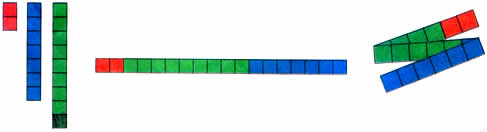Thursday , July 7 2022# NCERT 5th Class (CBSE) Mathematics: The Four Operations And Their Application

### CHECK POINT

The average is always between the lowest and the highest numbers.

(b) Three friends have an average of 46 stamps each. How many stamps do they have in all?

The friends have 46 + 46 + 46 0r 46 × 3 = 138 stamps in all.

(c) Try this activity to find the average o 2, 9 and 7.

Use squared paper and colour three separate strip of 2, 9 and 7 in three different colours. Then cut them out and paste them together as one long strip. Fold and cut the strip into three equal parts. How many squares are there in each part?## SPEED, DISTANCE AND TIME

You must have often seen lightning before you heard its thunder. That is because the speed of light is much faster than the speed of sound.

Speed is calculated taking into account the distance travelled  and the time it takes to travel that distance.

Speed = Distance / Time ( S = D / T)

In everyday life, we need to calculate the speed of cars, trains, planes or even a person walking. If a car goes 320 km in 4 hours, its speed is:

320 / 4 = 80 km per hour (kmph)

*Light travels at 300000 km per second and sound travels at 343 meters per second.

## चुनौती हिमालय की 5th NCERT CBSE Hindi Rimjhim Ch 18

चुनौती हिमालय की 5th Class NCERT CBSE Hindi Book Rimjhim Chapter 18 प्रश्न: लद्दाख जम्मू-कश्मीर राज्य में …